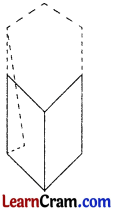# DAV Class 7 Maths Chapter 14 Brain Teasers Solutions

The DAV Class 7 Maths Book Solutions and DAV Class 7 Maths Chapter 14 Brain Teasers Solutions of Visualising Solids offer comprehensive answers to textbook questions.

## DAV Class 7 Maths Ch 14 Brain Teasers Solutions

Question 1.
A. Tick (✓) the correct option:represents a net of—
(a) cone
(b) cube
(c) cubiod
(d) cylinder
(d) cylinder

It represents a net of cylinder.
Hence, (d) is the correct option.

(ii) How many cubes are there in the given figure?(a) 13
(b) 14
(c) 15
(d) 16
(c) 15

15 cubes are there in the figure.
Hence, (c) is the correction option.

(iii) A tetrahedron has ___________ vertices.
(a) 2
(b) 3
(c) 4
(d) 5
(c) 4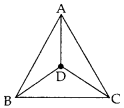A tetrahedron has four vertices A, B, C and D.
Hence, (c) is the correct

(iv) I have one square and four triangles. I am a
(a) cube
(b) pyramid
(c) prism
(d) cubiod
(b) pyramidA pyramid has one square and four triangles as shown in the given figure.
Hence, (b) is the correctc option.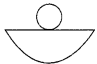can be folded into a:
(a) cone
(b) sphere
(c) cylinder
(d) circle
(a) cone

It can be folded into a cone.
Hence, (a) is the correct option.(i) Draw a net for a tetrahedron.
Nets for a tetrahedron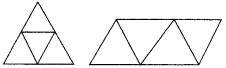(ii) Can this be a net for a die? Why or why not?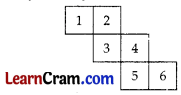No, When the net is folded, it will look like the figure given alongside, in which sum of opposite face digits is not equal to seven (7).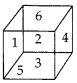(iii) Complete the net for making a cube.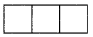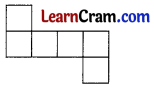(iv) Two cubes of edge 2 cm each are placed side to side to form a cuboid. Draw an oblique sketch for the resulting figure.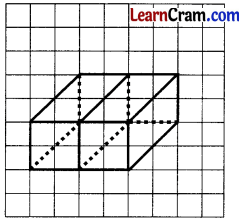(v) What is the difference between a cube and a cuboid?
All faces of a cube are squares and all faces of a cuboid are rectangles.

## DAV Class 7 Maths Chapter 14 HOTS

Question 1.
Which figure will be opposite to the triangle when the net is folded into a cube?will be opposite to the triangle when the net is folded into a cube.Question 2.
Draw the top view and front view of a bolt with a hexagonal head and a hexagonal nut.